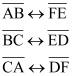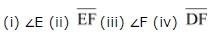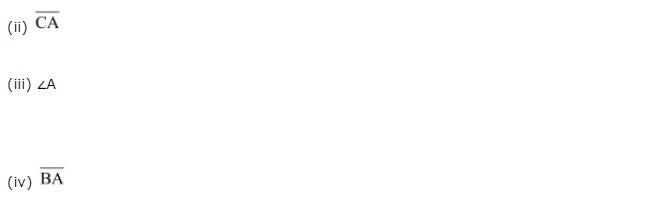# NCERT Solutions class 7 Maths Chapter-7 Exercise 7.1

## NCERT Solutions class 7 Maths Chapter-7 Congruence of Triangles

### Solutions of Chapter Congruence of Triangles Exercise-7.1

Question 1:
Complete the following statements:
(a) Two line segments are congruent if __________.
(b) Among two congruent angles, one has a measure of 70°; the measure of the other angle is __________.
(c) When we write ∠A = ∠ B, we actually mean __________.
(a) They have the same length
(b) 70°
(c) m ∠A = m ∠B

Question 2:
Give any two real-life examples for congruent shapes.
(i) Sheets of same letter pad
(ii) Biscuits in the same packet

Question 3:
If /ABC ≅ /FED under the correspondence ABC ↔ FED, write all the Corresponding congruent parts of the triangles.
If these triangles are congruent, then the corresponding angles and sides will be equal to each other.
∠A ↔ ∠F
∠B ↔ ∠E
∠C ↔ ∠DQuestion 4:
If /DEF ≅ /BCA, write the part(s) of /BCA that correspond to